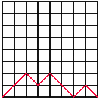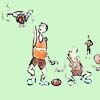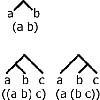# Search by Topic

#### Resources tagged with Patterned numbers similar to Modular Fractions:

Filter by: Content type:
Age range:
Challenge level:

### There are 13 results

Broad Topics > Numbers and the Number System > Patterned numbers### Magic Squares II

##### Age 14 to 18

An article which gives an account of some properties of magic squares.### Harmonic Triangle

##### Age 14 to 16 Challenge Level:

Can you see how to build a harmonic triangle? Can you work out the next two rows?### Pinned Squares

##### Age 14 to 16 Challenge Level:

What is the total number of squares that can be made on a 5 by 5 geoboard?### Odd Differences

##### Age 14 to 16 Challenge Level:

The diagram illustrates the formula: 1 + 3 + 5 + ... + (2n - 1) = n² Use the diagram to show that any odd number is the difference of two squares.### Triangles Within Pentagons

##### Age 14 to 16 Challenge Level:

Show that all pentagonal numbers are one third of a triangular number.### Magic Squares

##### Age 14 to 18

An account of some magic squares and their properties and and how to construct them for yourself.### Rolling Coins

##### Age 14 to 16 Challenge Level:

A blue coin rolls round two yellow coins which touch. The coins are the same size. How many revolutions does the blue coin make when it rolls all the way round the yellow coins? Investigate for a. . . .### How Old Am I?

##### Age 14 to 16 Challenge Level:

In 15 years' time my age will be the square of my age 15 years ago. Can you work out my age, and when I had other special birthdays?### Back to Basics

##### Age 14 to 16 Challenge Level:

Find b where 3723(base 10) = 123(base b).### Sixty-seven Squared

##### Age 16 to 18 Challenge Level:

Evaluate these powers of 67. What do you notice? Can you convince someone what the answer would be to (a million sixes followed by a 7) squared?##### Age 14 to 16 Challenge Level:

A walk is made up of diagonal steps from left to right, starting at the origin and ending on the x-axis. How many paths are there for 4 steps, for 6 steps, for 8 steps?### One Basket or Group Photo

##### Age 7 to 18 Challenge Level:

Libby Jared helped to set up NRICH and this is one of her favourite problems. It's a problem suitable for a wide age range and best tackled practically.### Counting Binary Ops

##### Age 14 to 16 Challenge Level:

How many ways can the terms in an ordered list be combined by repeating a single binary operation. Show that for 4 terms there are 5 cases and find the number of cases for 5 terms and 6 terms.